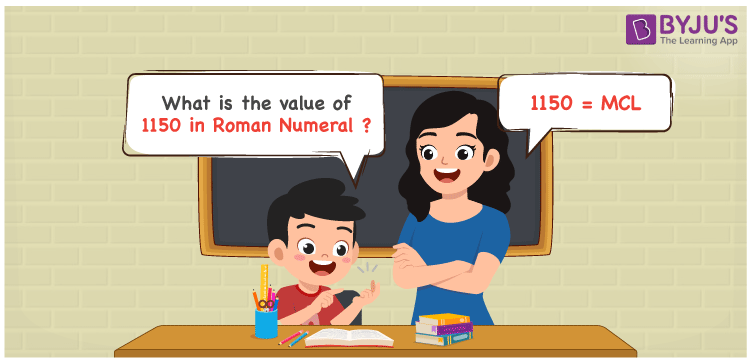# 1150 in Roman Numerals

1150 in Roman Numerals is MCL. Any Roman numeral uses only the 7 primary symbols which are I, V, X, L, C, D, and M, Where M represents 1000, C represents 100 and L represents 50. MCL represents the number 1150 which is obtained by the addition rule of Roman Numerals. What are Roman numerals? can be referred for more details.

 Number Roman Numeral 1150 MCL

## How to Write 1150 in Roman Numerals?To convert 1150 in Roman Numerals, we need to represent 1150 as the sum of the fundamental symbols. 1150 can be broken down as Sum of 1000 with 100 and 10. Since all these 3 numbers have fundamental symbols, the Roman numeral representation of 1150 is MCL.

Let us understand it with Numerical representation.

1150 = 1000 + 100 + 50

1150 = M + C + L

1150 = MCL

## Video Lesson on Roman Numerals## Frequently Asked Questions on 1150 in Roman Numerals

Q1

### How to write the number 1150 in Roman Numerals?

1150 in Roman Numerals is written as MCL.
Q2

### Does MCL represent Roman Numerals?

Yes. MCL represents Roman Numerals and it is the number 1150
Q3

### What is the year 1150 in Roman numerals?

The year 1150 in Roman Numerals is MCL.